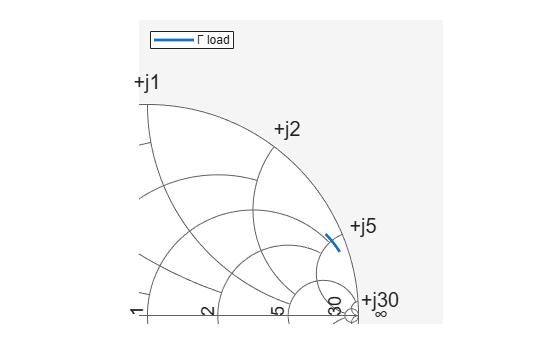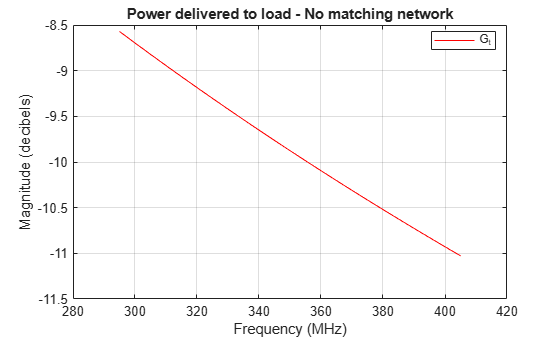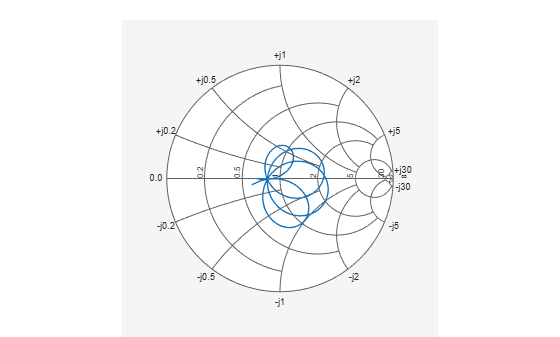Documentation

## Designing Broadband Matching Networks (Part 1: Antenna)

This example shows how to design a broadband matching network between a resistive source and inductive load using optimization with direct search methods.

In any system that uses RF circuits, a matching network is necessary to transfer the maximum amount of power between a source and a load. In most systems, such as wireless devices, there is a bandwidth of operation specified. As a result the purpose of the matching network is to provide maximum power transfer over a range of frequencies. While the L section matching approach (conjugate match), guarantees maximum power transfer, it does so only at a single frequency.Figure 1: Impedance matching of an antenna to a source

### Specify Frequency and Impedance

You are building a matching network with a bandpass response, so specify the center frequency and the bandwidth of match.

```fc = 350e6; % Center Frequency (Hz) BW = 110e6; % Bandwidth (Hz) ```

Here you specify the source impedance, the reference impedance and the load resistance. In this example the load `Zl` is modeled as a series R-L circuit. You could instead measure the impedance of the load and use that directly.

```Zs = 50; % Source impedance (ohm) Z0 = 50; % Reference impedance (ohm) Rl = 40; % Load resistance (ohm) L = 12e-8; % Load inductance (Henry) ```

Define the number of frequency points to use for analysis and set up the frequency vector.

```nfreq = 256; % Number of frequency points fLower = fc - (BW/2); % Lower band edge fUpper = fc + (BW/2); % Upper band edge freq = linspace(fLower,fUpper,nfreq); % Frequency array for analysis w = 2*pi*freq; % Frequency (radians/sec) ```

### Understand Load Behavior using Reflection Coefficient and Power Gain

You then use two simple expressions for calculating the load reflection coefficient and the power gain. This corresponds to directly connecting the source to the antenna input terminals i.e. in Figure 1 there is no matching network.

```Xl = w*L; % Reactance (ohm) Zl = Rl + 1i*Xl; % Load impedance (ohm) GammaL = (Zl - Z0)./(Zl + Z0); % Load reflection coefficient unmatchedGt = 10*log10(1 - abs(GammaL).^2); % Power delivered to load ```

Use the `smithplot` function to plot the variation in the load reflection coefficient with frequency. An input reflection coefficient closer to center of the Smith chart means a better matching performance. This plot shows that the load reflection coefficient is far away from this point. Therefore, there is an impedance mismatch.

```figure hs = smithplot(GammaL); hs.LegendLabels = {'#Gamma load'}; ```You can confirm this mismatch by plotting the transducer gain as a function of frequency.

```plot(freq.*1e-6,unmatchedGt,'r') grid on title('Power delivered to load - No matching network') xlabel('Frequency (MHz)') ylabel('Magnitude (decibels)') legend('G_t','Location','Best') ```As the plot shows, there is approximately 10 dB power loss around the desired region of operation (295 - 405 MHz). As a result, the antenna needs a matching network that operates over a 110 MHz bandwidth that is centered at 350 MHz.

### Design the Matching Network

The matching network must operate between 295 MHz and 405 MHz, so you choose a bandpass topology for the matching network which is shown here.

Type - I: Series LC first element followed by shunt LCFigure 2: Matching network topology

The approach is to design an odd order 0.5 dB Chebyshev lowpass prototype and then apply a lowpass to bandpass transformation  to obtain the initial design for the matching network shown in figure 2. You now need to enter the order desired and the associated coefficients. This is a single match problem , i.e. the source is purely resistive while load is a combination of R and L, so you can begin by choosing a five element prototype network.

```N = 5; % Order of matching network LCproto = [1.7058 1.2296 2.5408 1.2296 1.7058]; % Lowpass prototype values (Normalized) wU = 2*pi*fUpper; % Upper band edge wL = 2*pi*fLower; % Lower band edge w0 = sqrt(wL*wU); % Geometric mean ```

Use the `lcladder` object to build the bandpass tee matching network. The impedance and frequency transformations are included for denormalization purposes. Please note that the topology demands a bandpass tee prototype that begins with a series inductor. If the topology chosen is an LC bandpass pi then you would begin with shunt C for the lowpass prototype.

```Lvals = zeros(N,1); Cvals = zeros(N,1); Lvals(1:2:end) = LCproto(1:2:end).*Zs./(wU-wL); % Series L's (H) Cvals(1:2:end) = (wU-wL)./(Zs.*(w0^2).*LCproto(1:2:end)); % Series C's (F) Lvals(2:2:end) = ((wU-wL)*Zs)./((w0^2).*LCproto(2:2:end)); % Shunt L's (H) Cvals(2:2:end) = LCproto(2:2:end)./((wU-wL).*Zs); % Shunt C's (F) % Create the matching network matchingNW = lcladder('bandpasstee',Lvals,Cvals); % Copy initial values for comparison L_initial = Lvals; ```

### Optimize the Designed Matching Network

There are several points to consider prior to the optimization

• Objective function - The objective function can be built in different ways depending on the problem at hand. For this example, the objective function is shown in the file below.

• Choice of cost function - The cost function is the function we would like to minimize (maximize) to achieve near optimal performance. There could be several ways to choose the cost function. One obvious choice is the input reflection coefficient, gammaIn. In this example we have chosen to minimize the average reflection coefficient in the passband.

• Optimization variables - In this case it is a vector of values, for the specific elements to optimize in the matching network.

• Optimization method - A direct search based technique, the MATLAB® function `fminsearch`, is used in this example to perform the optimization.

• Number of iterations/function evaluations - Set the maximum number of iterations and function evaluations to perform, so as to tradeoff between speed and quality of match.

The objective function used during the optimization process by `fminsearch` is shown here.

```type('antennaMatchObjectiveFun.m') ```
```function output = antennaMatchObjectiveFun(matchingNW,Lvalues,freq,ZL,Z0) %ANTENNAMATCHOBJECTIVEFUN is the objective function used by the example % Designing Broadband Matching Networks (Part I: Antenna), which can be % found in broadband_match_antenna.m. % % OUTPUT = ANTENNAMATCHOBJECTIVEFUN(MATCHINGNW,LVALUES,FREQ,Z0) % returns the current value of the objective function stored in OUTPUT % evaluated after updating the inductor values in the object, MATCHINGNW. % The inductor values are stored in the variable LVALUES. % % ANTENNAMATCHOBJECTIVEFUN is an objective function of RF Toolbox demo: % Designing Broadband Matching Networks (Part I: Antenna) % Copyright 2008-2015 The MathWorks, Inc. % Ensure positive element values if any(Lvalues <= 0) output = Inf; return end % Update the element values in the matching network matchingNW.Inductances(1) = Lvalues(1); matchingNW.Inductances(end) = Lvalues(end); % Perform analysis on tuned matching network S = sparameters(matchingNW,freq,Z0); % Calculate input reflection coefficient 'gammaIn' gIn = gammain(S,ZL); % Cost function output = mean(abs(gIn)); % Other possible choices for objective function could be : - % output = max(abs(gIn)); % output = -1*mean(Gt_pass); % Animate hs = smithplot(gIn); % l.LegendLabels = 'Optimizing #Gamma in'; drawnow ```

There are several ways to choose the cost function and some options are shown within the objective function above (in comments). The optimization variables are the first and last inductors, L1 and L5 respectively. The element values are stored in the variable `L_Optimized`.

```niter = 125; options = optimset('Display','iter','MaxIter',niter); % Set options structure L_Optimized = [Lvals(1) Lvals(end)]; L_Optimized = ... fminsearch(@(L_Optimized)antennaMatchObjectiveFun(matchingNW, ... L_Optimized,freq,Zl,Z0),L_Optimized,options); ```
``` Iteration Func-count min f(x) Procedure 0 1 0.933981 1 3 0.933981 initial simplex 2 5 0.920321 expand 3 7 0.911351 expand 4 9 0.853251 expand 5 11 0.730432 expand 6 13 0.526433 reflect 7 15 0.526433 contract inside 8 17 0.421086 reflect 9 19 0.421086 contract inside 10 20 0.421086 reflect 11 22 0.421086 contract inside 12 24 0.421086 contract inside 13 26 0.339941 expand 14 27 0.339941 reflect 15 29 0.285288 reflect 16 31 0.285288 contract inside 17 32 0.285288 reflect 18 34 0.283533 reflect 19 36 0.283533 contract inside 20 38 0.278945 contract inside 21 40 0.278134 reflect 22 41 0.278134 reflect 23 43 0.276368 contract inside 24 45 0.275793 contract inside 25 47 0.275646 contract inside 26 49 0.275509 reflect 27 51 0.275292 contract inside 28 52 0.275292 reflect 29 54 0.275292 contract inside 30 56 0.275292 contract inside Optimization terminated: the current x satisfies the termination criteria using OPTIONS.TolX of 1.000000e-04 and F(X) satisfies the convergence criteria using OPTIONS.TolFun of 1.000000e-04 ```### Update the Matching Network Elements with Optimal Values

When the optimization routine stops, the optimized element values are stored in `L_Optimized`. The following code updates the input and output matching network with these values.

```matchingNW.Inductances(1) = L_Optimized(1); % Update the matching network inductor L1 matchingNW.Inductances(end) = L_Optimized(end); % Update the matching network inductor L5 ```

### Analyze and Display Optimization Results

Compare and plot the input reflection coefficient of the matched and unmatched results.

```hold all hs = smithplot(GammaL); hs.LegendLabels = {'#Gamma in (Matched)','#Gamma in (Unmatched)'}; hold off ```The optimized matching network improves the performance of the circuit. In the passband (295 MHz to 405 MHz), the input reflection coefficient is closer to the center of the Smith chart.

Plot the power delivered to load for both the matched and unmatched system.

```S = sparameters(matchingNW,freq,Z0); matchedGt = powergain(S,Zs,Zl,'Gt'); figure; plot(freq*1e-6,matchedGt) hold all plot(freq*1e-6,unmatchedGt,'r') grid on hold off title('Power delivered to load') legend('Optimized network','No matching network','Location','Best'); ```The power delivered to the load is approximately 1 dB down for the optimized matching network.

### Display Optimized Element Values

The following code shows the initial and optimized values for inductors L1 and L5.

```L1_Initial = L_initial(1) L1_Optimized = L_Optimized(1) ```
```L1_Initial = 1.2340e-07 L1_Optimized = 1.2111e-07 ```
```L5_Initial = L_initial(end) L5_Optimized = L_Optimized(end) ```
```L5_Initial = 1.2340e-07 L5_Optimized = 1.7557e-09 ```

There are a few things to consider when setting up an optimization:

• Choosing a different objective function would change the result.

• You can use advanced direct search optimization functions such as `patternsearch` and `simulannealband` in your optimization, but you must have the Global Optimization Toolbox installed to access them.

A Low noise amplifier design example is covered in the second example Designing Broadband Matching Networks (Part 2: Amplifier).

### References

 RF Circuit Design, Theory and Applications, Reinhold Ludwig and P. Bretchko, pp 229-239,Prentice Hall, 2000.

 Microwave Engineering, David M. Pozar, 2nd ed., John Wiley and Sons, 1999.

 Broadband Direct-Coupled and Matching RF networks, Thomas R. Cuthbert, pp 31-33, TRCPEP, 1999.